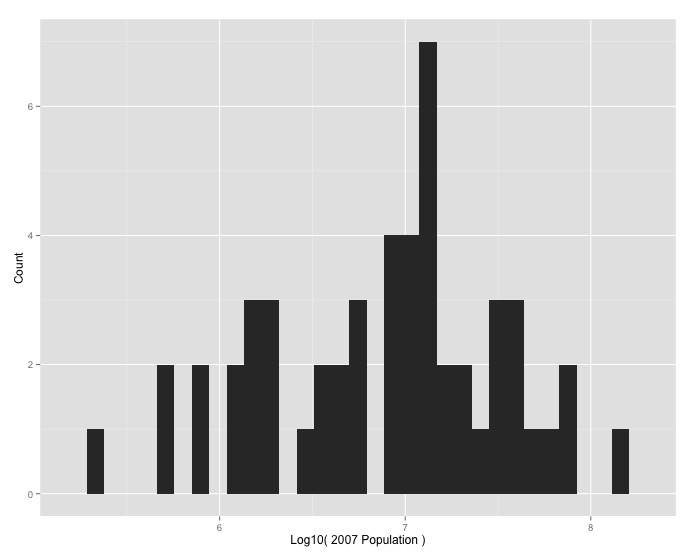# ggplot2 Basics

## Overview

Teaching: 10 min
Exercises: 5 min
Questions
• What is the grammar of graphics established by `ggplot2`?

• How does `ggplot2` generate graphics through layers?

Objectives
• To understand `ggplot2` aesthetic mapping of layers

• To generate graphics using `ggplot2`

• `ggplot2` implements the notion that every plot is drawn by adding layers on top of one another - just like as if you were drawing.

Suppose you were asked to draw a graph. Before drawing, you would possibly have several questions on how to do it: 1. Which data should be mapped into the plot: is it the relationship between a categorical and a continuous variable, or that between two continuous variables; 2. Which geometrical representation should you use: a bar, a point or a density line? 3. You may also determine the appearance, the aesthetic, of the geometrical elements: are they going to be colored or filled? If they are a point, should it be a circle, a square or a triangle?

It is this reasoning from the Grammar of Graphics that is used to create plots in the `ggplot2` package.

Implement histogram for “How was the population of Africa distributed across the continent by country in 2007?”

## `ggplot` Shuffle Challenge

Rearrange the following code to generate a histogram.

``````geom_histogram() +
labs(x = "Log10( 2007 Population )", y = "Count")
ggplot(Africa, aes(log10(pop_2007))) +
``````

## Output Solution## Code Solution

``````ggplot(Africa, aes(log10(pop_2007))) +
geom_histogram() +
labs(x = "Log10( 2007 Population )", y = "Count")
``````

## Key Points

• Use `ggplot2` to visualize and analyze data.